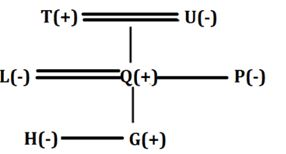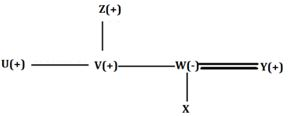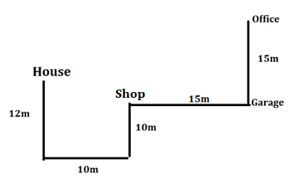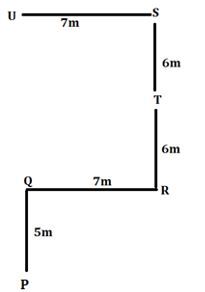Latest Banking jobs   »

# Reasoning Ability Quiz For IBPS Clerk/PO Prelims 2022- 11th August

Directions (1-5): Read the following arrangement of numbers, alphabets and symbols carefully and answer the questions based on it.

9 O 6 P & A 4 U S M # O 2 9 S A ? & # 2 Q 0 8 2 P @ 4 5 ! A 8 8 A \$

Q1. How many vowels are immediately followed by a number in the given arrangement?
(a) One
(b) Two
(c) Three
(d) Four
(e) More than four

Q2. If all the symbols are dropped then which element will be 6th from the left end after the rearrangement?
(a) U
(b) M
(c) 4
(d) A
(e) None of these

Q3. If all the numbers are dropped then which element will be 5th from the right end after the rearrangement?
(a) @
(b) Q
(c) P
(d) !
(e) None of these

Q4. What is the sum of 5th number from the left end and 3rd number from the right end in the given arrangement?
(a) 13
(b) 7
(c) 11
(d) 14
(e) None of these

Q5. How many numbers are immediately followed by vowel and immediately preceded by letter in the given arrangement?
(a) One
(b) Two
(c) Three
(d) Four
(e) More than four

Directions (6-8): answer the questions based on the information given below.
In a family, L is married to Q. U is the mother-in-law of L. U has two children and one of them is unmarried. P is the paternal aunt of G. T is the paternal grandfather of G who is the only son of L. H is child of Q. Q is a male.

Q6. How is H related to the daughter of U?
(a) Niece
(b) Nephew
(c) Either Niece or Nephew
(d) Son
(e) Daughter

Q7. Who is sister-in-law of P?
(a) L
(b) Q
(c) U
(d) T
(e) None of these

Q8. How many female members in the family?
(a) One
(b) Two
(c) Three
(d) Either two or three
(e) None of these

Directions (9-10): answer the questions based on the information given below.
Six members are there in a family having three generations and one married couple. U is the unmarried brother of V. W is the mother of X. V is the brother of W. Y is the son in law of Z who is male. X is not married. Z has only two sons.

Q9. Who is the father of V?
(a) D
(b) Z
(c) X
(d) Y
(e) None of these

Q10. Who is the husband of W?
(a) X
(b) Y
(c) U
(d) V
(e) None of these

Directions (11-12): answer the questions based on the information given below.
Pinky started her journey from her house to her office. She started moving towards south from her house and after moving 12m she took left turn and moved 10m. She again took left turn and walked 10m to stop at a shop. From there, she moves 15m to her right and stops at a garage. From there, she moves another 15m to her left and stops at her office.

Q11. What is the direction of garage with respect to her house?
(a) North-east
(b) North-west
(c) South-east
(d) South-west
(e) None of the above

Q12. What is the direction of her office with respect to shop?
(a) North-east
(b) North-west
(c) South-east
(d) South-west
(e) None of the above

Directions (13-15): answer the questions based on the information given below.
Point P is 5m south of point Q. Point Q is 7m west of point R. Point S is 6m north of point T. Point R is 6m south of point T. Point S is 7m east of point U.

Q13. What is the direction of point P with respect to point S?
(a) North-east
(b) North-west
(c) South-east
(d) South-west
(e) None of the above

Q14. What is the shortest distance between point U and point P?
(a) 15m
(b) 16m
(c) 17m
(d) 18m
(e) None of the above

Q15. What is the direction of point U with respect to point R?
(a) North-east
(b) North-west
(c) South-east
(d) South-west
(e) None of the above

Solutions

S1. Ans. (d)
Sol. Given series – 9 O 6 P & A 4 U S M # O 2 9 S A ? & # 2 Q 0 8 2 P @ 4 5 ! A 8 8 A \$
Hence, there are four vowels which are immediately followed by a number.

S2. Ans. (c)
Sol. After operation – 9 O 6 P A 4 U S M O 2 9 S A 2 Q 0 8 2 P 4 5 A 8 8 A
Hence, 6th from the left end – 4

S3. Ans. (a)
Sol. After operation – O P & A U S M # O S A ? & # Q P @ ! A A \$
So, 5th from the right end – @

S4. Ans. (d)
Sol. 5th number from the left end = 9, 3rd number from the right end = 5,
So, required sum = 9 + 5 = 14.

S5. Ans. (a)
Sol. Given series – 9 O 6 P & A 4 U S M # O 2 9 S A ? & # 2 Q 0 8 2 P @ 4 5 ! A 8 8 A \$
Hence, there is one number which is immediately followed by vowel and preceded by letter in the given arrangement.

Solution (6-8):
Sol.S6. Ans. (a)
S7. Ans. (a)
S8. Ans. (e)

Solution (9-10):
Sol.S9. Ans. (b)
S10. Ans. (b)

Solution (11-12):
Sol.S11. Ans. (c)
S12. Ans. (a)

Solution (13-15):
Sol.S13. Ans. (d)
S14. Ans. (c)
S15. Ans. (b)#### Congratulations!Download Hindu Review of October 2021: Free PDF